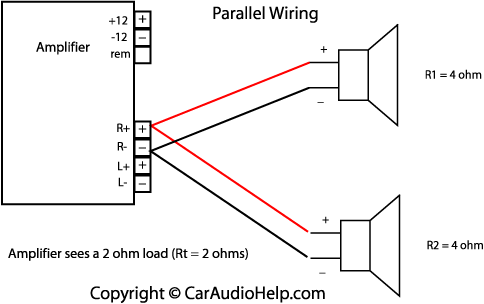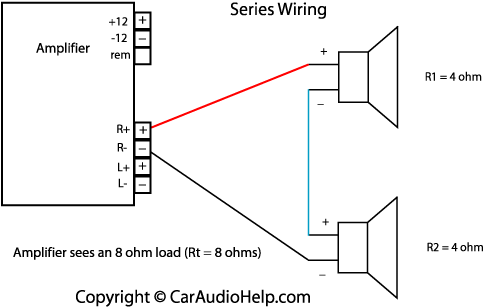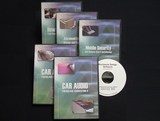# Ohm's Law in Car Audio

Ohm's law is the most basic and most useful electrical equation. It's used frequently in car audio installation on both the power input and the power output side. Simply stated Ohm's law is:

E=I*R

Where E is voltage measured in volts, I is current measured in amperes (amps) and R is resistance measured in ohms. Memorize this equation. You'll use it A LOT in car audio. For example, if you need to figure out the current (amps) moving through a 12 volt circuit and you know the resistance of the circuit is 4 ohms, the equation would look like this:

E = 12volts
I = unknown
R = 4 ohms

I = E/R or I = 12/4 which is I = 3 amps

Another useful equation to know is the power equation:

P = E*I (power equals voltage multiplied by current or watts = volts * amps). From this we can substitute Ohm's law for any values we don't know. For instance if we need to know power but we only have amperage (I) and resistance (R) then we could substitute I*R in the power equation (because according to Ohm's law E=I*R) and get P = I*R*I.

## Wiring

There are two ways to wire electrical components. In parallel or in series (or a combination of the two). Both are important to understand, especially when properly hooking up speakers to amplifiers.

### Parallel Wiring

Parallel wiring is connecting components to a source so that they share the same voltage. To put that in a useful way, it would be connecting all of the speaker positive terminals to the positive terminal of the amplifier and connecting all of the speaker negative terminals to the negative terminal of the amplifier.This increases the work load on the amplifier because more current will need to be supplied to this lower resistance (impedance). Parallel resistances (in this case 4 ohm speakers) will combine according to this equation:

1/Rt = 1/R1 + 1/R2 + 1/R3...

Where Rt is the total resistance and R1, R2 and R3 are the individual resistances. For our example Rt will be the resistance at the amplifier's speaker output terminals and R1, R2 and R3 will be the resistances of the individual speakers. If we connect (2) four ohm speakers (R1 and R2) in parallel to an amplifier the total resistance will be:

1/Rt = 1/R1 + 1/R2 or 1/Rt = 1/4 + 1/4 or 1/Rt = 1/2

Inverting the equation we get Rt = 2 ohms.

Similarly if we connect (3) four ohm speakers (R1, R2, and R3) we will get:

1/Rt = 1/R1 + 1/R2 + 1/R3 or 1/Rt = 1/4 + 1/4 + 1/4 or 1/Rt = 3/4
Inverting the equation we get Rt = 4/3 or 1.33 ohms.

## Series Wiring

Series wiring is connecting components to a source so that they share the same current. To put that in a useful way, it would be connecting the amplifier's positive terminal to the positive terminal of the first speaker and then connecting the negative terminal of the first speaker to the positive terminal of the second speaker and so on. The final speaker in the chain will have it's negative terminal connected to the negative terminal of the amplifier.This decreases the work load on the amplifier because less current will need to be supplied to this higher resistance (impedance). Series resistances (in this case 4 ohm speakers) will combine according to this equation:

Rt = R1 + R2 + R3...

Where Rt is the total resistance andR1, R2 and R3 are the individual resistances. For our example Rt will be the resistance at the amplifier's speaker outputs andR1, R2 and R3 will be the resistances of the individual speakers. If we connect (2) four ohm speakers (R1 and R2) in series to an amplifier the total resistance will be:

Rt = R1 + R2 or Rt = 4 + 4 or Rt = 8 ohms

Similarly if we connect (3) four ohm speakers (R1, R2, and R3) we will get:

Rt = R1 + R2 + R3 or Rt = 4 + 4 + 4 or Rt = 12 ohmsThe Car Audio Help DVD catalog includes five different videos covering many areas of car audio installation and custom fabrication. Topics range from basic system installation (head units, amplifiers, speakers, etc.) and mobile security (car alarms and remote start) to subwoofer box design and fiberglass fabrication. If you're interested in custom fabrication and car audio installation be sure to check out what we have to offer.

Nextl Page ---> Electronic Components